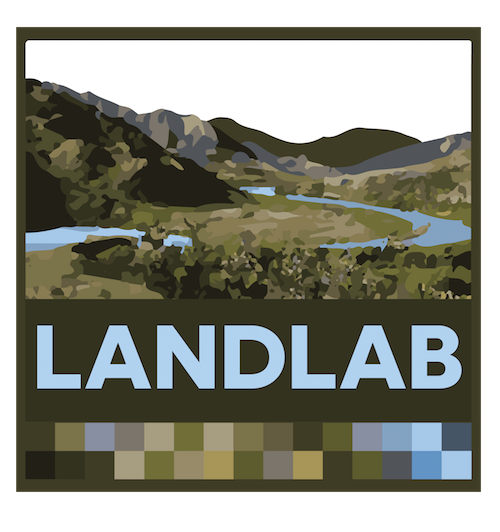# Reuse a published regional Landlab shallow landslide model to explore changes in forest cover at a subcatchment within the study area¶

### You will explore how to reuse model code and data in a subregion within a larger region where the model was developed¶

• Load data from a regional Landlab landslide model (Strauch et al., 2018) developed for the North Cascades National Park, WA USA, published on HydroShare.
• Define a geographic subset (Thunder Creek watershed) within the study region.
• Explore landslide probability sensitivity to fire by adjusting the cohesion parameter for Thunder Creek.
• Save results to a new HydroShare resource.

The shallow landslide model you will is based on a spatially distributed Monte Carlo solution of the infinite slope stability model. Detailes of the model and the study site are described in Strauch et al. (2018). Please see the end of this Notebook for Acknowledgements and Citation information.## To run this notebook:¶

Click in each shaded cell below and "shift + enter" to run each code block. Alternatively, you can run groups of cells by clicking "Cell" on the menu above and selecting your run options from the pull-down menu. This is also where you can clear outputs from previous runs.

If an error occurs, click on Kernal and Restart and Clear Outputs in the menu above.

## 1. Introduction¶

1.1 Infinite Slope Factor of Safety Equation

This equation predicts the ratio of stabilizing to destabilizing forces on a hillslope plane. The properties are assumed to represent an infinte plane, neglecting the boundary conditions around the landslide location. When FS<1, the slope is instable.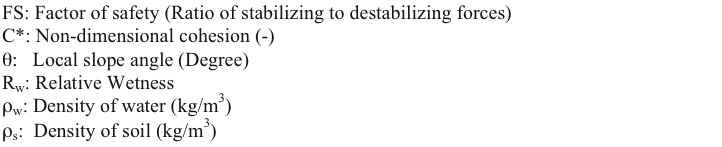C* can be calculated by the ratio of the sum of root cohesion, Cr, and soil cohesion, Cs, to the product of soil depth, density, and gravity.Relative wetness is the ratio of depth of water subsurface flow above an impervious layer to the depth of soil.R: recharge rate to water table (m/d) T: soil transmissivity (m^2/d) a: specific catchment area (m).

1.2 Monte Carlo solution of the FS equation

Below the Monte Carlo approach used by Strauch et al. (2018) is illustrated in a figure. At each node of the model grid variables for soil and vegetation are generated from triangular distributions. Recharge to water table is obtained from hydrologic model simulations by selecting the largest daily value for each year. Probability of shallow landslide initiation is defined as the ratio of number of times FS<1 to the total sample size of FS calculations.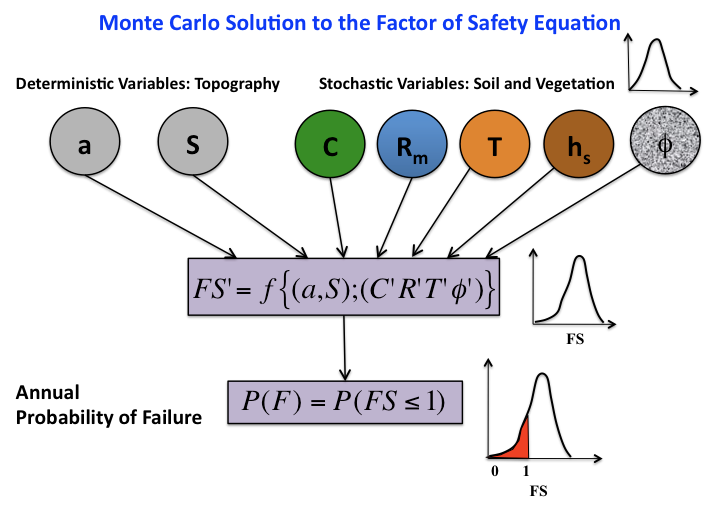### Module overview for a Landlab Modeling Toolkit Landslide Introduction¶

This Jupyter Notebook runs the Landlab LandslideProbability component on a 30-m digital elevation model (DEM) for Thunder Creek, a subwaterhsed in the larger study domain developed by Strauch et al. (2018), using data driven spatial recharge distribution as described in the paper (https://www.earth-surf-dynam.net/6/1/2018/).

To run a landslide demonstration using the paper data and approach we will:
1) Import data from North Cascades National Park (NOCA: study area)
2) Review data needed as input for the landslide model
3) Create a RasterModelGrid based on a 30-m DEM - subset to Thunder Creek watershed
4) Assign data fields used to calculate landslide probability
5) Specify recharge option as data driven spatial and access Python dictionaries to generate recharge distributions
6) Set Number of iterations to run Monte Carlo simulation
7) Run Landlab LandslideProbability component
8) Run the model again to simulate post-fire conditions,
9) Display and visualize results of stability analysis
10) Save Notebook and Results back to HydroShare

The estimated time to run this Notebook is 30-60 minutes with 20 minutes of computation time.

## 2.0 Data Science & Cyberinfrastructure Methods¶

Update or add libraries that are not installed on the CUAHSI JupyterHub server. At the time of publication, the Landlab library was dependent on a different numpy library than installed in the software environment. The code block below was added as an example of how to update a library to a new version and restart the notebook kernel. If this update is not needed, comment with a <#>. If other updates are needed, add them below.

In :
# comment this out if this update is not needed
#!conda update -y numpy
#from IPython.display import display_html
#display_html("<script>Jupyter.notebook.kernel.restart()</script>",raw=True)


### 2.1.1 Import Landlab components, functions for importing data, plotting tools, and HydroShare utilities¶

To run this notebook, we must import several libraries. The hs_utils library provides functions for interacting with HydroShare, including resource querying, dowloading. and creation. Additional libraries support the functions of Landlab. The CUAHSI JupyterHub server provides many Python packages and libraries, but to add additional libraries to your personal user space, use the cell below. To request an Installation to the server, visit https://github.com/hydroshare/hydroshare-jupyterhub, create a New Issue, and add the label 'Installation Request'.

### 2.1.2 Import Python libraries¶

In :
#Import standard Python utilities for calculating and plotting
import six
import os

import matplotlib as mpl
mpl.use('agg')
import matplotlib.pyplot as plt

import warnings
warnings.filterwarnings('ignore')

import numpy as np
import pandas as pd
import pickle as pickle
from datetime import datetime, timedelta
import geopandas as gpd

# Import Landlab libraries
from landlab.components import LandslideProbability
from landlab import imshow_grid_at_node
from landlab.io import write_esri_ascii
from landlab.plot import imshow_grid
from landlab import CORE_NODE, CLOSED_BOUNDARY

#Import utilities for importing and exporting to HydroShare
from hstools import hydroshare

# Import general tools
import time
from collections import defaultdict

st = time.time()

%matplotlib inline


### 2.1.3 Connection with HydroShare¶

After importing libraires, we now establish a secure connection with HydroShare by instantiating the hydroshare class that is defined within hs_utils. In addition to connecting with HydroShare, this command also sets and prints environment variables for several parameters that will be useful for saving work back to HydroShare.

In :
hs=hydroshare.hydroshare()
homedir=os.getcwd()
#homedir = os.path.join('/home/jovyan/work/notebooks/data', str(homeresid),str(homeresid),'data/contents/')
#print('This is a basic Unix folder structure.')
#print('Data will be loaded from and saved to:'+homedir)

The hs_utils library requires a secure connection to your HydroShare account.
Enter the HydroShare password for user 'drew': ········
Do you want to save this password for future use?
Note: the password will be stored in plain text (not recommended) [y/N]?n
Successfully established a connection with HydroShare


If you are curious about where the data is being downloaded, click on the Jupyter Notebook dashboard icon in upper rigth corner to see a File System view. The homedir directory location printed above is where you can find the data and contents you will download to a HydroShare JupyterHub server. At the end of this work session, you can migrate this data to the HydroShare iRods server as a Generic Resource.

### 2.2. Cyberinfrastructure methods¶

Strauch et al (2018) pre-processed the data for the North Cascades National Park Complex case study and is on HydroShare as Regional landslide hazard using Landlab - NOCA Data. Here the first task is find this resource on Hydrohsare. We will click on the link to see the published data repository on HydroShare and collect the resource ID of the data. The resource ID can be found in the "How to cite" box, and it will be the series of numbers and letters following "hs.". Here's the copied resource: http://dx.doi.org/10.4211/hs.a5b52c0e1493401a815f4e77b09d352b citation. Now we copy this ID and introduce it as "Data_ResourceID=" in the code below.

In :
Data_ResourceID='a5b52c0e1493401a815f4e77b09d352b'


We will execute the next cell to download data from HydroShare iRods database to your personal user space - this may take a few minutes.

In :
hs.getResourceFromHydroShare(Data_ResourceID)
data_folder = os.path.join(os.getcwd(), hs.getContentPath(Data_ResourceID), 'Data files')

Download Finished

Found the following file(s) associated with this HydroShare resource.
Data files
Driver and related codes
Results
These files are stored in a dictionary called hs.content for your convenience. To access a file, simply issue the following command where MY_FILE is one of the files listed above:
hs.content["MY_FILE"]
This HydroShare resource has been downloaded to: /home/jovyan/work/Downloads/370c288b61b84794b847ef85c4dd4ffb/370c288b61b84794b847ef85c4dd4ffb/data/contents/hs_downloads/a5b52c0e1493401a815f4e77b09d352b/a5b52c0e1493401a815f4e77b09d352b/data/contents/Data files


### 2.3. Review data needed as input for the landslide model¶

To view the code source for this component, visit Landlab on Github or Download the landslide_probability.py python file

Check the list of data inputs that the component needs.

In :
sorted(LandslideProbability.input_var_names)

Out:
['soil__density',
'soil__internal_friction_angle',
'soil__maximum_total_cohesion',
'soil__minimum_total_cohesion',
'soil__mode_total_cohesion',
'soil__saturated_hydraulic_conductivity',
'soil__thickness',
'soil__transmissivity',
'topographic__slope',
'topographic__specific_contributing_area']

Review the details of what each variable represents.

In :
LandslideProbability._var_doc

Out:
{'topographic__specific_contributing_area': 'specific contributing (upslope area/cell face ) that drains to node',
'topographic__slope': 'slope of surface at node represented by tan theta',
'soil__transmissivity': 'mode rate of water transmitted through a unit width of saturated soil - either provided or calculated with Ksat and soil depth',
'soil__saturated_hydraulic_conductivity': 'mode rate of water transmitted through soil - provided if transmissivity is NOT provided to calculate tranmissivity  with soil depth',
'soil__mode_total_cohesion': 'mode of combined root and soil cohesion at node',
'soil__minimum_total_cohesion': 'minimum of combined root and soil cohesion at node',
'soil__maximum_total_cohesion': 'maximum of combined root and soil cohesion at node',
'soil__internal_friction_angle': 'critical angle just before failure due to friction between particles',
'soil__density': 'wet bulk density of soil',
'soil__thickness': 'soil depth to restrictive layer',
'soil__mean_relative_wetness': 'Indicator of soil wetness; relative depth perched water table within the soil layer',
'landslide__probability_of_failure': 'number of times FS is <=1 out of number of iterations user selected',
'soil__probability_of_saturation': 'number of times relative wetness is >=1 out of number of iterations user selected'}

Check the units of each variable.

In :
LandslideProbability._var_units

Out:
{'topographic__specific_contributing_area': 'm',
'topographic__slope': 'tan theta',
'soil__transmissivity': 'm2/day',
'soil__saturated_hydraulic_conductivity': 'm/day',
'soil__mode_total_cohesion': 'Pa or kg/m-s2',
'soil__minimum_total_cohesion': 'Pa or kg/m-s2',
'soil__maximum_total_cohesion': 'Pa or kg/m-s2',
'soil__internal_friction_angle': 'degrees',
'soil__density': 'kg/m3',
'soil__thickness': 'm',
'soil__mean_relative_wetness': 'None',
'landslide__probability_of_failure': 'None',
'soil__probability_of_saturation': 'None'}

Now we will establish a RasterModelGrid based on a DEM for assigning our variables to. Nodes are the center point of grid cells or pixels that are 30 m by 30 m in this example.

### 2.4. Create a watershed subset using a regional RasterModelGrid¶

The shapefile that you will download is a GIS point file with a table containing a grid_code for each 30m DEM cell in the Thunder Creek watershed. We import this table to generate a list of locations we want to keep "Open" as active nodes in this Landlab application for Thunder Creek.

Here we will establish the watershed domain for our modeling study. In step 2.2.2, we have downloaded the DEM of the entire NOCA study region of Strauch et al. (2018) as well as other input data. This includes NoData resource that was created using a mask of Thunder Creek will be downladed and used to set no data nodes as inactive nodes (e.g., -9999). This step will establish boundary conditions. In your final run when you output modeled probability of landslide initiation, you will notice gaps in the model results at some ridge tops and peaks. This results from excluding glaciated areas and bedrock.
This might take a few minutes as the park is large (2,757 km2).

#### 2.4.1 Get shapefile of ThunderCreek watershed¶

The shapefile table contains Landlab Gridcode value and Albers Conical X and Y values, spatial projection is WGS84.

In :
Data_ResourceID='8bf8de77227c4dcba4816dbe15e55687'
hs.getResourceFromHydroShare(Data_ResourceID)

Download Finished

Found the following file(s) associated with this HydroShare resource.
Thunder_node_id_WGS.cpg
Thunder_node_id_WGS.dbf
Thunder_node_id_WGS_meta.xml
Thunder_node_id_WGS.prj
Thunder_node_id_WGS_resmap.xml
Thunder_node_id_WGS.sbn
Thunder_node_id_WGS.sbx
Thunder_node_id_WGS.shp
Thunder_node_id_WGS.shx
These files are stored in a dictionary called hs.content for your convenience. To access a file, simply issue the following command where MY_FILE is one of the files listed above:
hs.content["MY_FILE"]
In :
Node_path = os.path.join(hs.getContentPath(Data_ResourceID), 'Thunder_node_id_WGS.shp')
NodeID_shpfile=gpd.GeoDataFrame.from_file(Node_path)


Print a sample portion (bottom) of the table

• Col 1 = FID or POINTID
• Col 2 = landlab grid code for NOCA region
• Col 3 Point_X - the Alber Conical Latitude (original dataset)
• Col 4 Point_Y - the Alber Conical Longitude (original dataset)
• Geometry of lat/lon in WGS coordinate system of this GIS point file for launching in HydroShareGIS App
In :
NodeID_shpfile.tail()

Out:
POINTID GRID_CODE POINT_X POINT_Y geometry
306808 0 2348058 -1841730.0 3076320.0 POINT (-120.9517781910061 48.49903226527576)
306809 0 2348059 -1841700.0 3076320.0 POINT (-120.9513901735801 48.49910301141747)
306810 0 2348060 -1841670.0 3076320.0 POINT (-120.9510021553025 48.49917375650436)
306811 0 2348061 -1841640.0 3076320.0 POINT (-120.9506141361734 48.49924450053641)
306812 0 2345876 -1841640.0 3076290.0 POINT (-120.9505098563902 48.49898126887325)

Make an array using Grid_Code column to develop a node based mask. No output from this command.

In :
filtercriteria = np.array(NodeID_shpfile.GRID_CODE)


#### Load spatial landslide model inputs from ASCII textfile (ArcGIS raster conversion) into Landlab grid¶

Create Landlab RasterModelGrid using DEM grid with elevation - this takes approximately 60 sec for the North Cascades National Park (NOCA).

In :
(grid, z) = read_esri_ascii(data_folder+'/elevation.txt',name='topographic__elevation')
grid.at_node.keys()

Out:
dict_keys(['topographic__elevation'])

The original NOCA dem dataset has nodes closed outside of NOCA. All nodes insides of NOCA are open. To close all all nodes except those inside Thunder Creek:

• Close all nodes in the landlab RasterModelGrid.
• Open the Landlab RasterModelGrid only within the Thunder Creek watershed.
• Assign Core Nodes (these are the only nodes where computation will be executed).

Plot the elevation grid of NOCA

In :
imshow_grid(grid, 'topographic__elevation', limits=(0, 3000))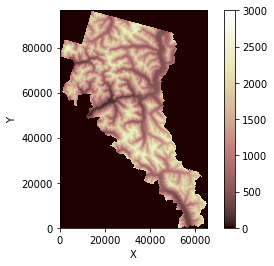Plot where the watershed is witin NOCA and zoom in.

In :
grid.status_at_node[grid.nodes.flatten()] = CLOSED_BOUNDARY
grid.status_at_node[filtercriteria] = CORE_NODE

In :
# In case the DEM has no data values inside the subset area, set boundary conditions closed.
grid.set_nodata_nodes_to_closed(grid.at_node['topographic__elevation'], -9999)

In :
fig = plt.figure('Limit NOCA computational nodes to Thunder Creek extent')
xticks = np.arange(-0.1, 0.8, 0.4)
ax1.xaxis.set_visible(False)
imshow_grid(grid, 'topographic__elevation', limits=(0, 3000),plot_name='NOCA extent',
allow_colorbar=False,color_for_closed='white')
ax2.xaxis.set_visible(False)
imshow_grid(grid, 'topographic__elevation', limits=(0, 3000),plot_name='Thunder Creek extent',color_for_closed='white')
plt.xlim(25000, 55000)
plt.ylim(30000, 55000)

Out:
(30000.0, 55000.0)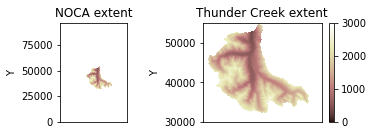Confirm the size of the grid, nodes located every 30 m.

In :
grid.number_of_nodes

Out:
7029145

Confirm the size of the core nodes where we'll run our model, a subset of the nodes for the watershed.

In :
grid.number_of_core_nodes

Out:
306798

### 2.5. Attach data (e.g., soil and vegetation variables) to the Landlab rastermodelgrid¶

This will be used to calculate shallow landslide probability and set boundary conditions. THe data we attach in this step was downloaded in Step 2.2.2.

#### For each input below¶

1. Load data from ascii text file
2. Add this data as node variable to the Thunder Creek grid
3. Set boundary conditions

For the entire NOCA extent, this takes ~60 sec to load each file using the CUAHSI JupyterHub server.

In :
(grid1, slope) = read_esri_ascii(data_folder+'/slope_tang17d.txt')
grid.set_nodata_nodes_to_closed(grid.at_node['topographic__slope'], -9999)
grid.set_nodata_nodes_to_closed(grid.at_node['topographic__slope'], 0.0)

In :
print(np.max(grid.at_node['topographic__slope'][grid.core_nodes]))
print(np.min(grid.at_node['topographic__slope'][grid.core_nodes]))

4.430756
0.3057313


In :
(grid1, ca) = read_esri_ascii(data_folder+'/cont_area.txt')
grid.set_nodata_nodes_to_closed(grid.at_node['topographic__specific_contributing_area'], -9999)


In :
(grid1, T) = read_esri_ascii(data_folder+'/transmis.txt')
grid.set_nodata_nodes_to_closed(grid.at_node['soil__transmissivity'], -9999)


#### 2.5.4 Load cohesion (mode, min, and max)¶

This takes ~3 minutes because 3 cohesion fields are provided to create more flexibility in how cohesion is distributed on the landscape with different vegetation.

In :
(grid1, C) = read_esri_ascii(data_folder+'/cohesion_mode.txt')
C[C == 0.0] = 1.0  # ensure minimum is >0 Pa for use in distributions generation
grid.set_nodata_nodes_to_closed(grid.at_node['soil__mode_total_cohesion'], -9999)

grid.set_nodata_nodes_to_closed(grid.at_node['soil__minimum_total_cohesion'], -9999)

grid.set_nodata_nodes_to_closed(grid.at_node['soil__maximum_total_cohesion'], -9999)


#### 2.5.5 Load internal angle of friction¶

In :
(grid1, phi) = read_esri_ascii(data_folder+'/frict_angle.txt')
grid.set_nodata_nodes_to_closed(grid.at_node['soil__internal_friction_angle'], -9999)


#### 2.5.6 Set soil density values¶

In this example, we assign all nodes a constant value.

In :
grid['node']['soil__density'] = 2000*np.ones(grid.number_of_nodes)


In :
(grid1, hs2) = read_esri_ascii(data_folder+'/soil_depth.txt')
grid.set_nodata_nodes_to_closed(grid.at_node['soil__thickness'], -9999)


#### 2.5.8 Load observed landslide inventory. Class 1-5 are landslides, 8 is no landslide mapped for later plotting¶

In :
(grid1, slides) = read_esri_ascii(data_folder+'/landslide_type.txt')

Out:
array([-9999., -9999., -9999., ..., -9999., -9999., -9999.])

### 2.6. Specify recharge option as data driven spatial and access Python dictionaries to generate recharge distributions¶

Recharge in this model represents the annual maximum recharge in mm/day generated within the upslope contributing area of each model element. This corresponds to the wettest conditions expected annually, which would provide the highest pore-water pressure in a year. Details of this approach can be found in Strauch et al. (2018).

#### 2.6.1 Select recharge method from the component¶

In :
distribution = 'data_driven_spatial'


#### 2.6.2 Load pre-processed routed flows dictionaries¶

These contain HSD_id and fractional drainage at each node and recharge dictionaries. HSD is the Hydrologic Source Domain, which is the VIC hydrologic model data in this case study at ~5x6 km2 grid size. The 'pickle' utility loads existing dictionaries.

In :
# dict of node id (key) and HSD_ids (values)
# dict of node id (key) and fractions (values)
# dict of HSD id (key) with arrays of recharge (values)


#### 2.6.3 Combine dictionaries into ordered parameters¶

This sequence of parameters is required for data driven spatial distribution in the component. Recharge is this model is unique to each node represented by an array.

In :
HSD_inputs = [HSD_dict, HSD_id_dict, fract_dict]


### 2.7. Set Number of iterations to run Monte Carlo simulation¶

The landslide component employes the infinite slope model to calculate factor-of-safety index values using a Monte Carlo simulation, which randomly selects input values from parameter distributions. You can specify the number of Monte Carlo samples, but the default is 250. The larger the Monte Carlos sample size, the longer the program runs, but the more precise the probability of failure results become. Strauch et al. (2018) sampled 3,000 times for each parameter in each model grid.

In :
iterations = 250


## 3. Results¶

### 3.1. Run the Landlab LandslideProbability Component in Thunder Creek for pre-fire conditions¶

#### 3.1.1 Initialize Pre-fire¶

To run the landslide model, we first instantiate the LandslideProbability component with the above parameters, as well as the grid and number of samples we specified before. Instantiation creates an instance of a class called LS_prob.

No outputs are generated by this command as it is setting up the recharge and instantiating the component.

In :
LS_prob = LandslideProbability(grid,
number_of_iterations=iterations,
groudwater__recharge_distribution=distribution,
groudwater__recharge_HSD_inputs=HSD_inputs)


#### 3.1.2 Run the Pre-fire model¶

Once the component has been instantiated, we generate outputs from running the component by calling the component's 'calculate_landslide_probability' method using the class instance (e.g., LS_prob). The cell below runs the model; in the following section we will assessing the results. These calculations will take a few minutes given the size of the modeling domain represented by core nodes.

In :
LS_prob.calculate_landslide_probability()
print('Landslide probability successfully calculated')

Landslide probability successfully calculated


What is the maximum probabilty of failure we found?

In :
np.max(grid.at_node['landslide__probability_of_failure'][grid.core_nodes])

Out:
1.0

The outputs of landslide model simulation are:

In :
sorted(LS_prob.output_var_names)

Out:
['landslide__probability_of_failure',
'soil__mean_relative_wetness',
'soil__probability_of_saturation']

This simulation generates a probability value for each core node.

In :
grid.at_node['landslide__probability_of_failure']

Out:
array([ 0.,  0.,  0., ...,  0.,  0.,  0.])

This simulation generates a probability of saturation value for each core node as well.

In :
grid.at_node['soil__probability_of_saturation']

Out:
array([-9999., -9999., -9999., ..., -9999., -9999., -9999.])

-9999 means there is no data for that cell, it is a closed node.

### 3.2. Run the Landlab LandslideProbability Component in Thunder Creek for fire conditions¶

Make a 'grid_fire' version of 'grid' for which we will give post-fire cohesion parameter values 30% of the original cohesion. This is a crude estimation of the lowest root cohesion after tree removal based on a combined decay and regrowth model (e.g., Sidle, 1992).

Sidle, R. C. (1992), A theoretical model of the effects of timber harvesting on slope stability, Water Resour. Res., 28(7), 1897–1910.

#### 3.2.1 Make a Post-fire copy of the grid¶

In :
import copy
grid_fire=copy.deepcopy(grid)
grid_fire.at_node['soil__mode_total_cohesion']=grid.at_node['soil__mode_total_cohesion']*0.3
grid_fire.at_node['soil__minimum_total_cohesion']=grid.at_node['soil__minimum_total_cohesion']*0.3
grid_fire.at_node['soil__maximum_total_cohesion']=grid.at_node['soil__maximum_total_cohesion']*0.3


#### 3.2.2 Change the Post-fire cohesion values¶

In :
print("this is the highest mode value for coehsion before fire across the domain")
print(np.max(grid.at_node['soil__mode_total_cohesion'][grid.core_nodes]))
print("this is the lowest mode value for coehsion before fire across the domain")
print(np.min(grid.at_node['soil__mode_total_cohesion'][grid.core_nodes]))
print("this is the highest mode value for coehsion after fire across the domain")
print(np.max(grid_fire.at_node['soil__mode_total_cohesion'][grid.core_nodes]))
print("this is the lowest mode value for coehsion after fire across the domain")
print(np.min(grid_fire.at_node['soil__mode_total_cohesion'][grid.core_nodes]))

this is the highest mode value for coehsion before fire across the domain
10000.0
this is the lowest mode value for coehsion before fire across the domain
100.0
this is the highest mode value for coehsion after fire across the domain
3000.0
this is the lowest mode value for coehsion after fire across the domain
30.0


#### 3.2.3 Initialize the Post-fire model¶

Now we'll run the landslide component with the adjusted cohesion, everything else kept constant.

In :
LS_probFire = LandslideProbability(grid_fire,number_of_iterations=iterations,
groudwater__recharge_distribution=distribution,
groudwater__recharge_HSD_inputs=HSD_inputs)
print('Post-fire cohesion successfully instantiated')

Post-fire cohesion successfully instantiated


#### 3.2.4 Run the Post-fire model¶

In :
LS_probFire.calculate_landslide_probability()
print('Landslide probability successfully calculated')

Landslide probability successfully calculated

In :
np.max(grid_fire.at_node['landslide__probability_of_failure'][grid.core_nodes])

Out:
1.0

The outputs of landslide model simulation are:

In :
sorted(LS_probFire.output_var_names)

Out:
['landslide__probability_of_failure',
'soil__mean_relative_wetness',
'soil__probability_of_saturation']

This simulation generates a probability value for each core node.

In :
grid_fire.at_node['landslide__probability_of_failure']

Out:
array([ 0.,  0.,  0., ...,  0.,  0.,  0.])

### 3.3. Display and visualize results of stability analysis¶

Set plotting parameters

In :
mpl.rcParams['xtick.labelsize'] = 15
mpl.rcParams['ytick.labelsize'] = 15
mpl.rcParams['lines.linewidth'] = 1
mpl.rcParams['axes.labelsize'] = 18
mpl.rcParams['legend.fontsize'] = 15


Plot elevation

In :
plt.figure('Elevations from the DEM [m]')
imshow_grid_at_node(grid, 'topographic__elevation', cmap='terrain',
grid_units=('coordinates', 'coordinates'),
shrink=0.75, var_name='Elevation', var_units='m',color_for_closed='white')
plt.xlim(25000, 55000)
plt.ylim(30000, 55000)
plt.savefig('NOCA_elevation.png')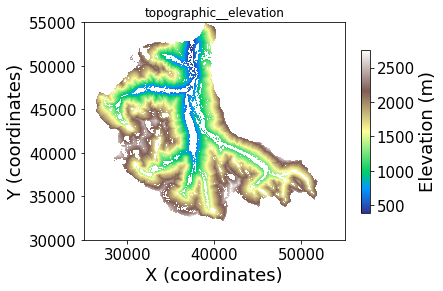Excluded areas from the analysis are shown in white, including outside the park and inside the park areas that are water bodies, snow, glaciers, wetlands, exposed bedrock, and slopes <= 17 degrees.

Plot slope overlaid with mapped landslide types. Takes about a few minutes.

In :
plt.figure('Landslides')
imshow_grid_at_node(grid, 'topographic__slope', cmap='pink',
grid_units=('coordinates', 'coordinates'), vmax=2.,
shrink=0.75, var_name='Slope', var_units='m/m',color_for_closed='white',)
imshow_grid_at_node(grid, overlay_landslide1, color_for_closed='None',
allow_colorbar=False, cmap='cool')
imshow_grid_at_node(grid, overlay_landslide2, color_for_closed='None',
allow_colorbar=False, cmap='autumn')
imshow_grid_at_node(grid, overlay_landslide3, color_for_closed='None',
allow_colorbar=False, cmap='winter')
imshow_grid_at_node(grid, overlay_landslide4, color_for_closed='None',
allow_colorbar=False,cmap='summer')
#plt.savefig('NOCA_Landslides_on_Slope.png')
plt.xlim(25000, 55000)
plt.ylim(30000, 55000)

Out:
(30000.0, 55000.0)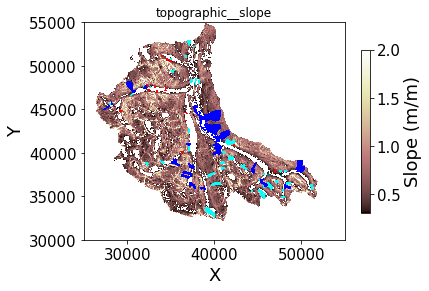Legend to mapped landslides: blue - debris avalanches, cyan - falls/topples, red - debris torrents, and green - slumps/creeps

Plot of soil depth (m)

In :
plt.figure('Soil Thickness')
imshow_grid_at_node(grid, 'soil__thickness', cmap='copper_r',
grid_units=('coordinates', 'coordinates'), shrink=0.75,
var_name='Soil Thickness', var_units='m', color_for_closed='white')
plt.xlim(25000, 55000)
plt.ylim(30000, 55000)
#plt.savefig('NOCA_SoilDepth.png')

Out:
(30000.0, 55000.0)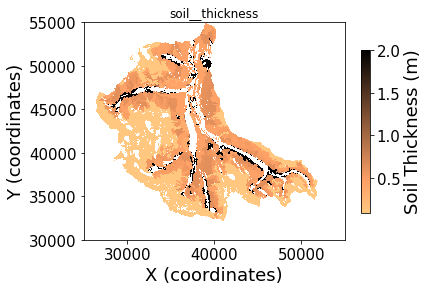Plot probability of saturation

In :
fig = plt.figure('Probability of Saturation')
xticks = np.arange(-0.1, 0.8, 0.4)
ax1.xaxis.set_visible(True)
imshow_grid(grid, 'soil__probability_of_saturation',cmap='YlGnBu',
limits=((0), (1)),plot_name='Pre-Fire',
allow_colorbar=False,grid_units=('coordinates', 'coordinates'), color_for_closed='white')
plt.xlim(25000, 55000)
plt.ylim(30000, 55000)
ax2.xaxis.set_visible(True)
ax2.yaxis.set_visible(False)
imshow_grid(grid_fire, 'soil__probability_of_saturation',cmap='YlGnBu',
limits=((0), (1)),plot_name='Post-Fire',grid_units=('coordinates', 'coordinates'), color_for_closed='white')
plt.xlim(25000, 55000)
plt.ylim(30000, 55000)

Out:
(30000.0, 55000.0)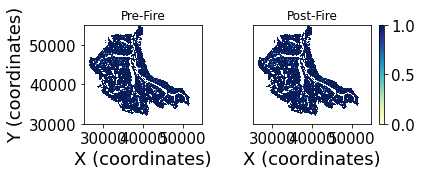This map shows the probability of saturation as high throughout much of the area because we modeled the annual maximum recharge, which is esssentially the worst case conditions that might lead to instability.

Plot probability of failure; Compare this with the elevation and slope maps.

In :
fig = plt.figure('Probability of Failure')
xticks = np.arange(-0.1, 0.8, 0.4)
#ax1.xaxis.set_visible(False)
imshow_grid(grid, 'landslide__probability_of_failure',cmap='OrRd',
limits=((0), (1)),plot_name='Pre-fire',
allow_colorbar=False,grid_units=('coordinates', 'coordinates'), color_for_closed='white')
plt.xlim(25000, 55000)
plt.ylim(30000, 55000)
ax2.yaxis.set_visible(False)
imshow_grid(grid_fire, 'landslide__probability_of_failure',cmap='OrRd',
limits=((0), (1)),plot_name='Post-fire',grid_units=('coordinates', 'coordinates'), color_for_closed='white')
plt.xlim(25000, 55000)
plt.ylim(30000, 55000)

#plt.savefig('Probability_of_Failure_Original_Fire.png')

Out:
(30000.0, 55000.0)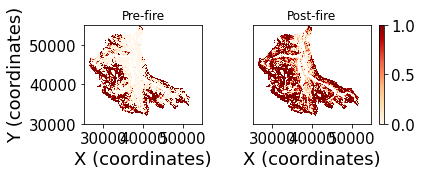The map of probability of failure shows higher probabilities in a post-fire scenario with reduced cohesion.

We can compare the range of probabilities before and after the simulated fire using a cumulative distribution plot.

In :
X1 = np.sort(np.array(grid.at_node['landslide__probability_of_failure'][grid.core_nodes]))
Y1 = np.arange(1, len(X1)+1)/len(X1)
X2 = np.sort(np.array(grid_fire.at_node['landslide__probability_of_failure'][grid.core_nodes]))
Y2 = np.arange(1, len(X2)+1)/len(X2)
fig = plt.figure('Empirical CDF of Probability of Failure')
plt.plot(X1,Y1, marker=' ', color='black', linewidth=2, label='Pre-fire')
plt.plot(X2,Y2, marker=' ', color='red', linewidth=2, linestyle='dashed', label='Post-fire')
plt.xlabel('Probability of Failure')
plt.ylabel('Fraction of Area')
plt.legend()

Out:
<matplotlib.legend.Legend at 0x7fefcd28c510>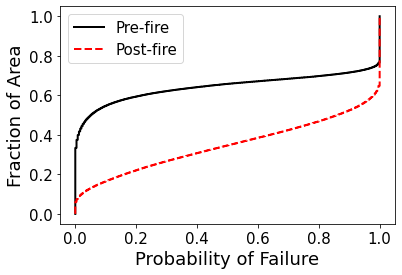We can interpret this figure as a higher fraction of the landscape has a higher probability of failure (red) after fire than before fire (black).

To review the fields assigned to the grid, simply execute the following command.

In :
grid.at_node

Out:
{'topographic__elevation': array([-9999., -9999., -9999., ..., -9999., -9999., -9999.]),
'topographic__slope': array([-9999., -9999., -9999., ..., -9999., -9999., -9999.]),
'topographic__specific_contributing_area': array([-9999., -9999., -9999., ..., -9999., -9999., -9999.]),
'soil__transmissivity': array([-9999., -9999., -9999., ..., -9999., -9999., -9999.]),
'soil__mode_total_cohesion': array([-9999., -9999., -9999., ..., -9999., -9999., -9999.]),
'soil__minimum_total_cohesion': array([-9999., -9999., -9999., ..., -9999., -9999., -9999.]),
'soil__maximum_total_cohesion': array([-9999., -9999., -9999., ..., -9999., -9999., -9999.]),
'soil__internal_friction_angle': array([-9999., -9999., -9999., ..., -9999., -9999., -9999.]),
'soil__density': array([ 2000.,  2000.,  2000., ...,  2000.,  2000.,  2000.]),
'soil__thickness': array([-9999., -9999., -9999., ..., -9999., -9999., -9999.]),
'landslides': array([-9999., -9999., -9999., ..., -9999., -9999., -9999.]),
'soil__saturated_hydraulic_conductivity': array([ 0.,  0.,  0., ...,  0.,  0.,  0.]),
'soil__mean_relative_wetness': array([ 0.,  0.,  0., ...,  0.,  0.,  0.]),
'landslide__probability_of_failure': array([ 0.,  0.,  0., ...,  0.,  0.,  0.]),
'soil__probability_of_saturation': array([-9999., -9999., -9999., ..., -9999., -9999., -9999.])}

Export data from model run: FS probability, mean Reletive wetness, probability of saturation

In :
import pandas as pd
core_nodes = grid.core_nodes
data_extracted = {'Prob_fail_std': np.array(
grid.at_node['landslide__probability_of_failure'][grid.core_nodes]),
'mean_RW_std': np.array(grid.at_node['soil__mean_relative_wetness']
[grid.core_nodes]),'prob_sat_std': np.array(
grid.at_node['soil__probability_of_saturation'][grid.core_nodes]),
'Prob_fail_fire': np.array(
grid_fire.at_node['landslide__probability_of_failure'][grid_fire.core_nodes]),
'mean_RW_fire': np.array(grid_fire.at_node['soil__mean_relative_wetness']
[grid_fire.core_nodes]),'prob_sat_fire': np.array(
grid_fire.at_node['soil__probability_of_saturation'][grid_fire.core_nodes])}
df.to_csv('Landslide_std_fire.csv')


Make ascii files for raster creation in GIS

In :
write_esri_ascii('prob_fail_std.txt',grid,names='landslide__probability_of_failure')
write_esri_ascii('mean_RW_std.txt',grid,names='soil__mean_relative_wetness')
write_esri_ascii('prob_sat_std.txt',grid,names='soil__probability_of_saturation')
write_esri_ascii('prob_fail_fire.txt',grid_fire,names='landslide__probability_of_failure')
write_esri_ascii('mean_RW_fire.txt',grid_fire,names='soil__mean_relative_wetness')
write_esri_ascii('prob_sat_fire.txt',grid_fire,names='soil__probability_of_saturation')

Out:
['prob_sat_fire.txt']

How long did the code above take to run?

In :
print('Elapsed time is %3.2f seconds' % (time.time() - st))

Elapsed time is 1419.82 seconds


## 4.0. Save the results back into HydroShare¶

Using the hs_utils library, the results of the Geoprocessing steps above can be saved back into HydroShare. First, define all of the required metadata for resource creation, i.e. title, abstract, keywords, content files. In addition, we must define the type of resource that will be created, in this case genericresource.

Note: Make sure you save the notebook at this point, so that all notebook changes will be saved into the new HydroShare resource.

Option A : define the resource from which this "NEW" content has been derived. This is one method for tracking resource provenance.

Create list of files to save to HydroShare. Verify location and names.

In :
ThisNotebook='replicate_landslide_model_for_fire.ipynb' #check name for consistency
files=[os.path.join(homedir, ThisNotebook),
os.path.join(homedir, 'Landslide_std_fire.csv'),
os.path.join(homedir, 'prob_fail_std.txt'),
os.path.join(homedir, 'mean_RW_std.txt'),
os.path.join(homedir, 'prob_sat_std.txt'),
os.path.join(homedir, 'prob_fail_fire.txt'),
os.path.join(homedir, 'mean_RW_fire.txt'),
os.path.join(homedir, 'prob_sat_fire.txt')]

for f in files:
if not os.path.exists(f):
print('\n[ERROR] invalid path: %s' % f)

In :
help(hs)

Help on hydroshare in module hstools.hydroshare object:

class hydroshare(builtins.object)
|
|  Methods defined here:
|
|      Initialize self.  See help(type(self)) for accurate signature.
|
|      Adds content files to an existing hydroshare resource.
|
|      args:
|      -- resid: id of an existing hydroshare resource (str)
|      -- content: files paths to be added to resource (list)
|
|      returns:
|      -- None
|
|  createHydroShareResource(self, abstract, title, derivedFromId=None, keywords=[], resource_type='GenericResource', content_files=[], public=False)
|      Creates a hydroshare resource.
|
|      args:
|      -- abstract: abstract for resource (str, required)
|      -- title: title of resource (str, required)
|      -- derivedFromId: id of parent hydroshare resource (str, default=>None)
|      -- keywords: list of subject keywords (list, default=>[])
|      -- resource_type: type of resource to create (str, default=>
|                                                   'GenericResource')
|      -- content_files: data to save as resource content (list, default=>[])
|      -- public: resource sharing status (bool, default=>False)
|
|      returns:
|      -- None
|
|  getContentFiles(self, resourceid)
|      Gets the content files for a resource that exists on the
|         Jupyter Server
|
|      args:
|      -- resourceid: the id of the hydroshare resource
|
|      returns:
|      -- {content file name: path}
|
|  getContentPath(self, resourceid)
|      Gets the server path of a resources content files.
|
|      args:
|      -- resourceid: the id of the hydroshare resource
|
|      returns:
|      -- server path the the resource content files
|
|  getResourceFromHydroShare(self, resourceid, destination='.')
|
|      args:
|      -- resourceid: id of the hydroshare resource (str)
|      -- destination: path to save resource, default
|
|      returns:
|      -- None
|
|      Gets metadata for a specified resource.
|
|      args:
|      -- resid: hydroshare resource id
|
|      returns:
|
|      Establishes a secure connection with hydroshare.
|
|      args:
|      -- email: email address associated with hydroshare
|
|      returns:
|      -- hydroshare api connection
|
|
|      args:
|      -- resourceid: the id of the resource that has been downloaded (str)
|
|      returns:
|      -- {content file name: path}
|
|  ----------------------------------------------------------------------
|  Data descriptors defined here:
|
|  __dict__
|      dictionary for instance variables (if defined)
|
|  __weakref__
|      list of weak references to the object (if defined)


In :
# Save the results back to HydroShare

# title for the new resource
title = 'Landslide Model run with Monte Carlo from NOCA Observatory - Thunder Creek with Fire'

# abstract for the new resource
abstract = 'This a reproducible demonstration of the landslide modeling results from eSurf paper: Strauch et al. (2018) '

# keywords for the new resource
keywords = ['landslide', 'climate', 'VIC','saturation','relative wetness','fire','Geohackweek']

# Hydroshare resource type
rtype = 'compositeresource'

# create the new resource
resource_id = hs.createHydroShareResource(abstract,
title,
keywords=keywords,
resource_type=rtype,
content_files=files,
public=False)

Resource Created Successfully

Resource id: 92cfc1ae46d543938facb98547f88eba
Successfully Added Content Files


### Acknowledgements¶This Notebooks serves as content for:

Bandaragoda, C. J., A. Castronova, E. Istanbulluoglu, R. Strauch, S. S. Nudurupati, J. Phuong, J. M. Adams, et al. “Enabling Collaborative Numerical Modeling in Earth Sciences Using Knowledge Infrastructure.” Environmental Modelling & Software, April 24, 2019. https://doi.org/10.1016/j.envsoft.2019.03.020.

and

Bandaragoda, C., A. M. Castronova, J. Phuong, E. Istanbulluoglu, S. S. Nudurupati, R. Strauch, N. Lyons, K. Barnhart (2019). Enabling Collaborative Numerical Modeling in Earth Sciences using Knowledge Infrastructure: Landlab Notebooks, HydroShare, http://www.hydroshare.org/resource/fdc3a06e6ad842abacfa5b896df73a76

This notebook was developed from code written by Ronda Strauch as part of her Ph.D. disseration at the University of Washington.

Use or citation of this notebook should also reference:

Strauch R., Istanbulluoglu E., Nudurupati S.S., Bandaragoda C., Gasparini N.M., and G.E. Tucker (2018). A hydro-climatological approach to predicting regional landslide probability using Landlab. Earth Surf. Dynam., 6, 1–26. https://www.earth-surf-dynam.net/6/1/2018/

In [ ]: